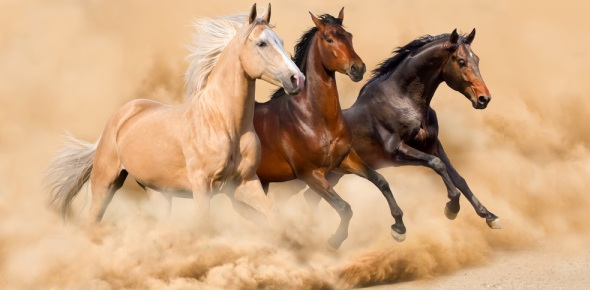# Motion And Forces

14 Questions | Total Attempts: 13Settings.

Related Topics
• 1.
With a pulley system when you pull down, the object goes down?
• A.

True

• B.

False

• 2.
When there is movement it is affected by
• A.

Mass, Weight and Shape

• B.

Surface, Direction and Mass

• C.

Mass, Force and Shape

• D.

Option 4

• 3.
What scientific tool would measure the distance a ball is launched from a launcher?
• A.

Meter Stick

• B.

Ruler

• C.

Scope

• D.

Option4

• 4.
Materials that would cause the most friction
• A.

Tile

• B.

Grass

• C.

Cotton

• D.

Rubber

• E.

Sand paper

• F.

Wax paper

• G.

Rubber

• H.

Dirt

• I.

Smooth surface

• J.

Gravel / sand

• 5.
A force is pushing or pulling on an object
• A.

True

• B.

False

• 6.
Materials that would cause the least friction:
• A.

Rubber

• B.

Tile

• C.

Grass

• D.

Wax paper

• E.

Sand

• F.

Smooth surface

• 7.
It is NOT important to investigate more than one time to ensure accurate (correct) results.
• A.

True

• B.

False

• 8.
How does the height of the ramp affect the distance an object will travel down the ramp?
• A.

The longer the ramp the longer the distance is

• B.

The higher the ramp the longer the distance

• C.

The shorter the ramp the faster it will go

• D.

Option4

• 9.
What investigation is being tested by using a spring scale and using it to pull a block across  different forces.
• A.

Speed

• B.

Amount of force

• C.

Weight

• D.

Distance

• 10.
Force and Mass relate to each other by the more mass an object has the more force it requires?
• A.

True

• B.

False

• 11.
Pull is away from you
• A.

True

• B.

False

• 12.
To push means to push away from you.
• A.

True

• B.

False